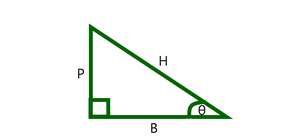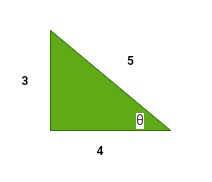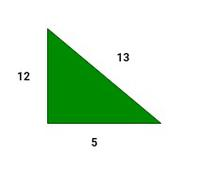Related Articles

# What are the six trigonometry functions?

• Last Updated : 03 Sep, 2021

Trigonometry can be defined as the branch of mathematics that determines and studies the relationships between the sides of a triangle and angles subtended by them. Trigonometry is basically used in the case of right-angled triangles. Trigonometric functions define the relationships between the 3 sides and the angles of a triangle. There are 6 trigonometric functions mainly. Before going into the study of the trigonometric functions we will learn about the 3 sides of a right-angled triangle.The three sides of a right-angled triangle are as follows,

• Base The side on which the angle θ lies is known as the base.
• Perpendicular It is the side opposite to the angle θ  in consideration.
• Hypotenuse It is the longest side in a right-angled triangle and opposite to the 90° angle.

### Trigonometric Functions

Trigonometry has 6 basic trigonometric functions, they are sine, cosine, tangent, cosecant, secant, and cotangent. Now let’s look into the trigonometric functions. The six trigonometric functions are as follows,

• sine It is represented as sin θ and is defined as the ratio of perpendicular and hypotenuse.
• cosine It is represented as cos θ and is defined as the ratio of base and hypotenuse.
• tangent It is represented as tan θ and is defined as the ratio of sine and cosine of an angle. Thus the definition of tangent comes out to be the ratio of perpendicular and base.
• cosecant It is the reciprocal of sin θ and is represented as cosec θ.
• secant It is the reciprocal of cos θ and is represented as sec θ.
• cotangent It is the reciprocal of tan θ and is represented as cot θ.

### What are the six trigonometry functions?

The six trigonometric functions have formulae for the right-angled triangles, the formulae help in identifying the lengths of the sides of a right-angled triangle, lets take a look at all those formulae,The below table shows the values of these functions at some standard angles,

Note: It is advised to remember the first 3 trigonometric functions and their values at these standard angles for ease of calculations.

### Sample Problems

Question 1: Evaluate sine, cosine, and tangent in the following figure.Solution:

GivenUsing the trigonometric formulas for sine, cosine and tangent,Question 2: In the same triangle evaluate secant, cosecant, and cotangent.

Solution:

As it is known the values of sine, cosine and tangent, we can easily calculate the required ratios.Question 3: Given, evaluate sin θ.cos θ.

Solution:Thus P=6, B=8

Using Pythagoras theorem,

H2=P2+B2

H2=36+64=100

Therefore, H =10

Now,Question 4: If, evaluate tan2θ.

Solution:

GivenThusQuestion 5: In the given triangle, verify sin2θ+cos2θ = 1Solution:

Given P=12, B=5, H=13

ThusHence verified.

Attention reader! All those who say programming isn’t for kids, just haven’t met the right mentors yet. Join the  Demo Class for First Step to Coding Coursespecifically designed for students of class 8 to 12.

The students will get to learn more about the world of programming in these free classes which will definitely help them in making a wise career choice in the future.

My Personal Notes arrow_drop_up